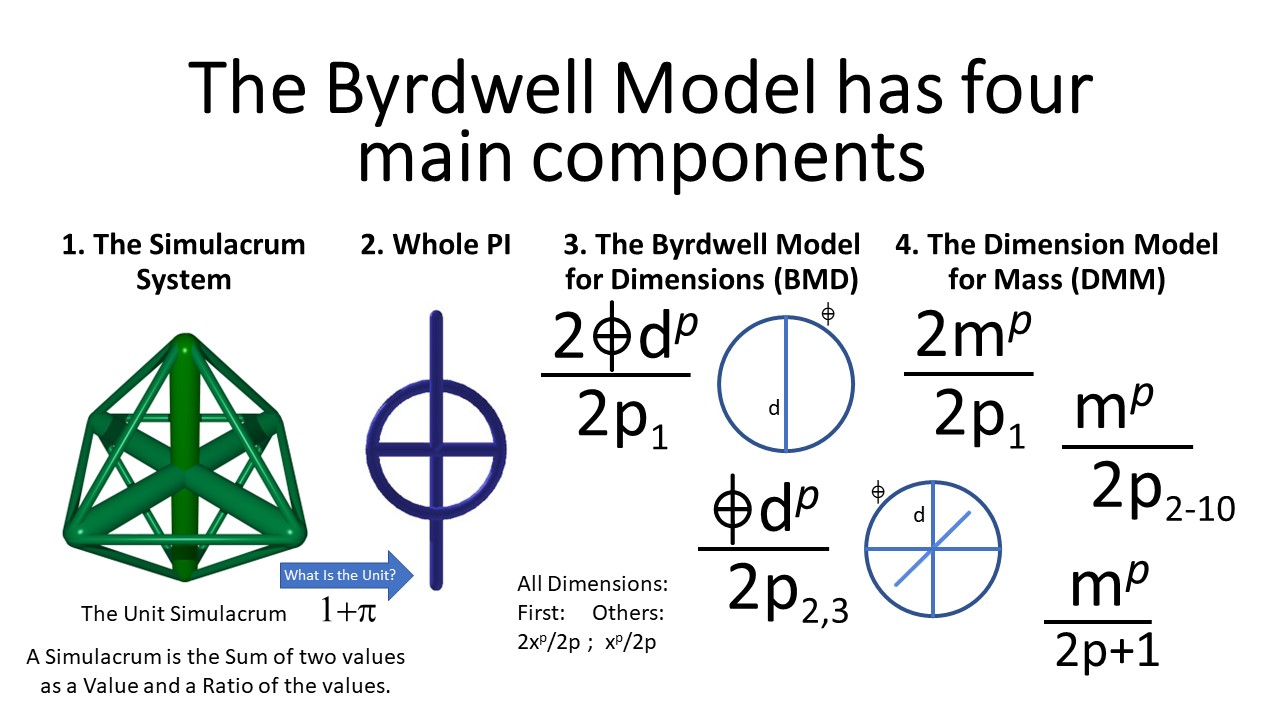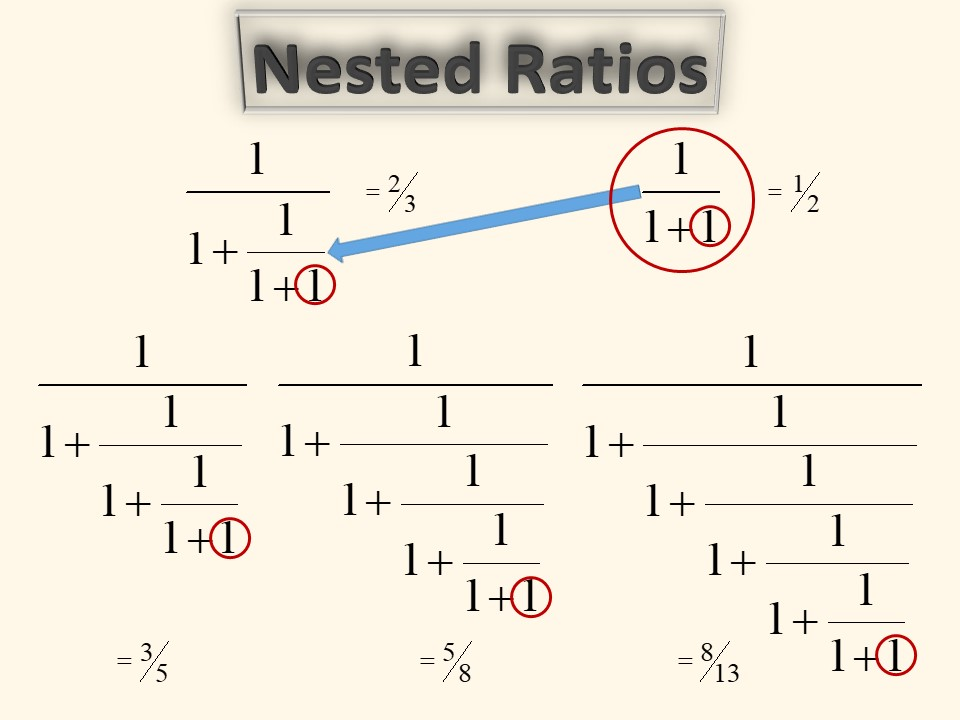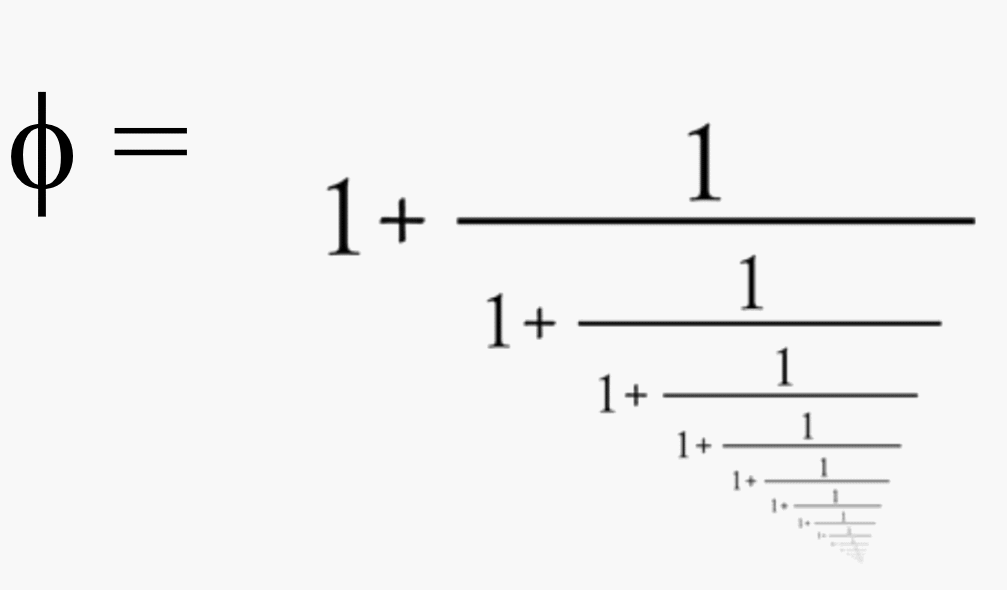# A FEW SIMPLE EQUATIONS TO UNDERSTAND THE WORLD.

See the common pattern behind Space and Mass!## See Dr. Byrdwell's Seminar

Part 1. Discovery of The Simulacrum System by Mass Spectrometry of Triacylglycerols (TAGs)
Part 2. The Simulacrum System and The Fibonacci Series, The Phi Ratio, and The Natural World
Part 3. The Simulacrum led to The Pi Paradox, Whole PI, and the Byrdwell Model for Dimensions (BMD)

## The Simulacrum is the equation behind all things.

The Simulacrum is the equation for The Matrix.
The Interpretation Matrix ties consciousness to symbols.
Everything is ratios of ratios. Ratios to definitions.

## The Unit Simulacrum leads directly to the Pi Simulacrum

The Unit Pi Simulacrum causes one to examine the nature of 'the unit'. This leads to The Pi Paradox.

Everything is Ratios of Ratios of Ratios to Ratios...
Everything is a Ratio of Thought to its Unit Definition

## The Unit Definition of Pi reveals The Pi Paradox

The Pi Paradox reveals the need for Whole PI.
Whole PI shows the common pattern behind Space, Mass, & The Periodic Table.## The Simulacrum leads to the Fibonacci Series and Golden Ratio

The inherent pattern in the Simulacrum shows that it  corresponds to Nature and natural processes.